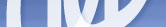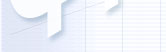Department and Science Statistical physics deals with systems with a large or infinite number of particles or modes. The systems investigated might be quantum or classical ones. but even the classical statistical physics uses methods of quantum field theory. Statistical physics is developed actively. While very expensive experiments are needed to test hypothesis of other fields of theoretical physics, the statistical physics problems are often presented free of charge. Indeed, a large set of phenomena in nature and industry concerning statistical physics is waiting for explaining. For example, the microscopic statistical theory of liquids have not created yet in spite of the existence of well developed statistical models of gases, plasma and solid bodies. A new class of phenomena investigated by statistical physics is in particular a chaos in non-linear systems, bifurcations, fractal systems. The statistical physics is developed now by a permanently growing number of theoretical physicists and scientific institutes. Maxwell, Einstein, Fermi, Feinmann, Landau, Fok, Heisenberg, Bogolubov contribute essentially to statistical physics in different times. Los Alamos National Lab, Sacly, Nordita, Max Plank Institutes and many other foundations are engaged in developing of statistical physics. The results of this efforts are essential. Statistical physics allow us to clarify and to describe quantitative superconductivity, superfluidity, turbulence, collective phenomena in solid bodies and plasma, structural properties of liquids. It generates the basis of the modern astrophysics, contributes to the liquid crystals physics and critical phenomena theory. Many experiments and based on a statistical description of systems, namely the scattering of cold ions, roentgen and visible light, correlation spectroscopy and so on. Mathematical methods applied in statistical physics are very numerous. Among them quantum mechanics and quantum-field methods, non-linear equation theory, various methods of mathematical physics. An important role is played by numerical simulations, such as Monte-Carlo calculations and molecular dynamics methods. In this way we can model real processes and obtain information inaccessible in other frameworks. Many great physicists were honoured with the Nobel prise for investigations in statistical physics, for example in theory of superconductivity, superfluidity, critical phenomena, liquid crystals and so on. There aren't Nobel laureates in our department yet:))) But most of our staff have the doctoral degree (D. Sc.). We have 2 Soros professors and 3 Soros docents. Our students and post-graduate student are often awarded to nominal research grants. The education we promise gives you very broad outlook in theoretical physics. There are lecture courses on stochastic theory and turbulence, critical phenomena, quantum phenomena in solid body and quantum liquids, theory of wave propagation in non-homogeneous mediums, polymer physics, condensation theory, liquid crystals etcetera. Our graduating students work in institutes of Russian Academy of Science or continue the scientific activity abroad.

The Department of Statistical Physic
Sain-Petersburg State University, Russia
Russian VersionMain fields of the scientific researchWave scattering and liquid crystals.The development of quantum - field theory to the statistical physics problems.The first order phase transitions theory.Electron properties of low dimension systems.